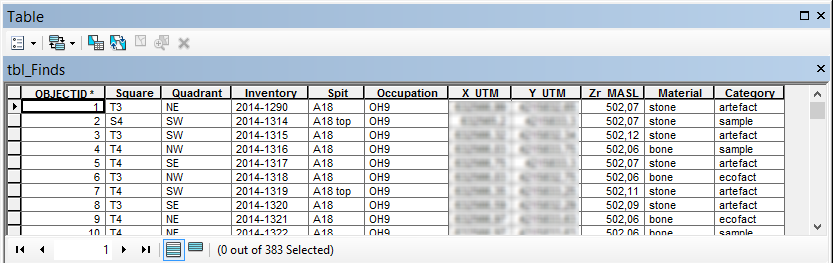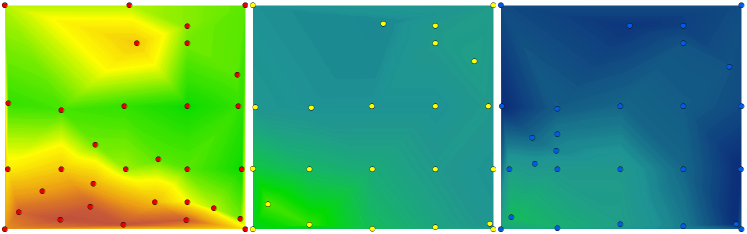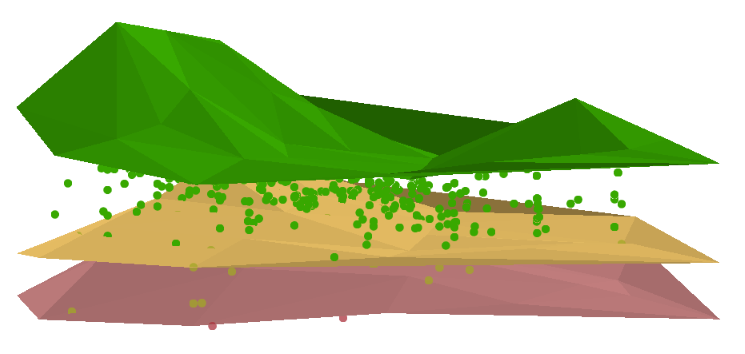# Modeling Archaeological Layers

1170
2
02-23-2015 05:01 PM
byEsri Esteemed Contributor
2 2 1,170

A few week ago my attention was drawn by the thread: https://community.esri.com/message/453147‌

The basic idea was to determine the soil layer of an archaeological find. In this thread 3D Analyst tools were used to obtain the result, but many steps were required to get there. I suggested to share some data to see if it was possible to make this process a little less manual and Armando was so kind to share not only part of this classified data, but also shared some insights in the wonders of archaeology.

Since the information is classified, the thread was made private. However, I do want to share the code and process that we worked. Maybe parts of it will be helpful for you or maybe you have suggestions to enhance the process.

Input data

The input data consists of two tables. One table holds the finds, with XYZ information:... and the other table hold topographic measurements and information on the soil type (the upper part of a soil layer):Outline of the process

A short description of the steps involved is:

• Explore the data and determine
• the extent of the xy coords of the find and topographic  information
• the number of soil layers (a list of the names of the levels: [OH9, OH10, OH11])
• Create points XYZ of the finds
• Create points (XYZ) of the topographic coords per soil layer
• Interpolate the topograhic points for each soil layer and convert to raster (3D Analyst)
• Perform a "Extract Multi Values to Points" (Spatial Analyst). This adds the values of the topographic rasters as attributes to the finds.
• Perform a loop to evaluate the attributes to find at what layer the find is located

A challenge

There was a challenge:

• The finds have an extent a little larger than the topographic information. The topographic information should be extended to match the extent of the findsExtending the topographic information

To extent the topographic information, I decided to create additional points at the corners of the data (the corners of the union of the finds and topographic extents). These corner points were added a Z value based on a weighted average of the 3 nearest points. Although this is an interpolation method, the influence of this error on the result is minimal.

Topographic points with additional corner points and the interpolation result (TIN, converted to raster):The result

The result of the analysis is the finds table converted to a z-aware featureclass enriched with the soil layer it was found in:The python code

Below the code that was used:

`#-------------------------------------------------------------------------------# Name:        archeo3D.py# Purpose:     analyze finds in layers## Author:      Xander## Created:     10-02-2015#-------------------------------------------------------------------------------import arcpyimport osimport mathdef main():    # input tables    tbl_finds = r"C:\Forum\Archeology\gdb\data.gdb\tbl_Finds"    tbl_topo = r"C:\Forum\Archeology\gdb\data.gdb\tbl_Topo"    fc_finds_name = "Find02" # output name    fld_out = "FindLayerOH"    # spatial reference and overwrite setting (True)    sr = arcpy.SpatialReference(23030) # ED_1950_UTM_Zone_30N    arcpy.env.overwriteOutput = True    # settings    cellsize = "CELLSIZE 0,01"    # workspace    ws = r"C:\Forum\Archeology\gdb\work.gdb"    ws_tin = r"C:\Forum\Archeology\TIN"    # output finds featureclass    fc_finds = os.path.join(ws, fc_finds_name)    # relevant fields for finds    # fld_find_layer = "Occupation"    fld_find_id = "Inventory"    fld_find_x = "X_UTM"    fld_find_y = "Y_UTM"    fld_find_z = "Zr_MASL"    # relevant fields for topo    fld_topo_id = "PointNumber"    fld_topo_layer = "OccupationTop"    fld_topo_x = "X_UTM"    fld_topo_y = "Y_UTM"    fld_topo_z = "Z_MASL"    # Check out extensions    arcpy.CheckOutExtension("Spatial")    arcpy.CheckOutExtension("3D")    # list with fields    flds_finds = [fld_find_id, fld_find_x, fld_find_y, fld_find_z]    flds_topo = [fld_topo_id, fld_topo_layer, fld_topo_x, fld_topo_y, fld_topo_z]    # create dictionary of finds    dct_finds = createDictionaryFromTable(tbl_finds, flds_finds)    # create dictionary of topo    dct_topo = createDictionaryFromTable(tbl_topo, flds_topo)    # get extent finds and topo and union    ext_finds = getExtentFromDct(dct_finds, flds_finds, fld_find_x, fld_find_y)    ext_topo = getExtentFromDct(dct_topo, flds_topo, fld_topo_x, fld_topo_y)    ext_tot = unionExtents(ext_finds, ext_topo)    # get soil layer list    lst_oh = getUniqueListValues(dct_topo, flds_topo.index(fld_topo_layer))    # create xyz featureclass for each layer with topo data    lst_xyz = []    for oh in lst_oh:        fc_xyz = os.path.join(ws, "xyz_{0}".format(oh))        lst_xyz.append(fc_xyz)        createXYZfeatureclass(fc_xyz, sr)        addFields2XYZfeatureclass(fc_xyz, flds_topo, tbl_topo)        fillXYZfeatureclassOH(fc_xyz, dct_topo, oh, fld_topo_layer, flds_topo,                            tbl_topo, fld_topo_x, fld_topo_y, fld_topo_z)        updateXYZwithExtentCornerPoints(fc_xyz, ext_tot)    # create the TIN's and convert to raster    lst_multiras = []    for oh in lst_oh:        fc_xyz = os.path.join(ws, "xyz_{0}".format(oh))        tin_xyz = os.path.join(ws_tin, "tin{0}".format(oh))        in_params = "{0} Shape.Z Mass_Points <None>".format(fc_xyz)        print in_params        arcpy.CreateTin_3d(out_tin=tin_xyz,spatial_reference=sr,                           in_features=in_params,                           constrained_delaunay="DELAUNAY")        # convert TIN to raster        ras_xyz = os.path.join(ws, "ras{0}".format(oh))        arcpy.TinRaster_3d(in_tin=tin_xyz, out_raster=ras_xyz, data_type="FLOAT",                           method="LINEAR", sample_distance=cellsize, z_factor="1")        lst_multiras.append([ras_xyz, "ras{0}".format(oh)])    # create xyz featureclass finds    createXYZfeatureclass(fc_finds, sr)    addFields2XYZfeatureclass(fc_finds, flds_finds, tbl_finds)    fillFindsfeatureclass(fc_finds, dct_finds, flds_finds, tbl_finds,                            fld_find_x, fld_find_y, fld_find_z)    # extract multi values to points    arcpy.gp.ExtractMultiValuesToPoints_sa(fc_finds, lst_multiras, "NONE")    # analyze attributes of finds featureclass    print "analyze attributes of finds featureclass"    flds_ohs = []    for lst in lst_multiras:        flds_ohs.append(lst)    # add output field    arcpy.AddField_management(fc_finds, fld_out, "TEXT", field_length=50)    # update the finds featureclass with the soil layer the find belongs to    flds = [fld_out, fld_find_z]    flds.extend(flds_ohs)    cnt = 0    with arcpy.da.UpdateCursor(fc_finds, flds) as curs:        for row in curs:            cnt += 1            lst_vals = list(row[2:])            lst_srt = sorted(lst_vals, reverse=True)            if row > lst_srt:                layer = "above upper layer"                layfound = None            else:                layfound = lst_srt                for laytop in lst_srt:                    if row < laytop:                        layfound = laytop            if not layfound == None:                i = lst_vals.index(layfound)                layer = flds[i+2]            row = layer            curs.updateRow(row)    # Check in extensions    arcpy.CheckOutExtension("Spatial")    arcpy.CheckOutExtension("3D")def updateXYZwithExtentCornerPoints(fc, ext):    """update XYZ featureclass with corner points of extent       use IDW to assign Z value to the corner points"""    flds = ('OID@', 'SHAPE@')    dct_xyz = {r: r for r in arcpy.da.SearchCursor(fc, flds)}    sr = arcpy.Describe(fc).spatialReference    # insert cursor    with arcpy.da.InsertCursor(fc, ('SHAPE@')) as curs:        # loop through corners        props = ["lowerLeft", "upperLeft", "upperRight", "lowerRight"]        for crnr in props:            dct_dist = {}            pnt = eval("ext.{0}".format(crnr))            for oid, pntg in dct_xyz.items():                dist = getDistance(pnt, pntg)                dct_dist[oid] = dist            # sort the dict on value            cnt = 0            lst_dist = []            lst_z = []            for oid, dist in sorted(dct_dist.items(), key=lambda x: x):                cnt += 1                lst_dist.append(dist)                lst_z.append(dct_xyz[oid].firstPoint.Z)                if cnt > 3:                    break            # distance weighted height for corners            sum_dist = sum(lst_dist)            z_res = 0            for i in range(len(lst_dist)):                d = lst_dist                z = lst_z                z_res += (d / sum_dist) * z            # create the point and insert it            pntz = arcpy.Point(pnt.X, pnt.Y, z_res)            curs.insertRow((pntz, ))def getDistance(pnt, pntg):    """Calculate the 2D distance between Point and PointGeometry"""    return math.hypot(pnt.X - pntg.firstPoint.X, pnt.Y - pntg.firstPoint.Y)def fillFindsfeatureclass(fc_finds, dct_finds, flds_finds, tbl_finds,                          fld_find_x, fld_find_y, fld_find_z):    """Fill the finds featureclass based on finds table, use Z aware points"""    flds = ["SHAPE@"]    flds.extend(flds_finds)    with arcpy.da.InsertCursor(fc_finds, flds) as curs:        for oid, lst_values in dct_finds.items():            pnt = arcpy.Point(lst_values[flds_finds.index(fld_find_x)],                              lst_values[flds_finds.index(fld_find_y)],                              lst_values[flds_finds.index(fld_find_z)])            lst_row = [pnt]            lst_row.extend(lst_values)            curs.insertRow(tuple(lst_row))def fillXYZfeatureclassOH(fc_xyz, dct_topo, oh, fld_topo_layer, flds_topo,                        tbl_topo, fld_topo_x, fld_topo_y, fld_topo_z):    """Fill the topo XYZ featureclass based on topo table       filter information on provided OH (soil layer)"""    flds = ["SHAPE@"]    flds.extend(flds_topo)    with arcpy.da.InsertCursor(fc_xyz, flds) as curs:        for oid, lst_values in dct_topo.items():            if lst_values[flds_topo.index(fld_topo_layer)] == oh:                pnt = arcpy.Point(lst_values[flds_topo.index(fld_topo_x)],                                  lst_values[flds_topo.index(fld_topo_y)],                                  lst_values[flds_topo.index(fld_topo_z)])                lst_row = [pnt]                lst_row.extend(lst_values)                curs.insertRow(tuple(lst_row))def addFields2XYZfeatureclass(fc, flds, tbl):    """Add field to FC based on list and extract settings for field from table"""    for fld_name in flds:        fld = arcpy.ListFields(tbl, wild_card=fld_name)        arcpy.AddField_management(fc, fld.name, fld.type, fld.precision,                                  fld.scale, fld.length)def createXYZfeatureclass(fc, sr):    """Create an empty Z-aware point featureclass"""    fc_ws, fc_name = os.path.split(fc)    arcpy.CreateFeatureclass_management(fc_ws, fc_name, "POINT", has_z="ENABLED",                                        spatial_reference=sr)def createDictionaryFromTable(tbl, flds_att):    """Create a dictionary with key is OID and values is a list of attributes"""    flds = ["OID@"]    flds.extend(flds_att)    dct = {}    with arcpy.da.SearchCursor(tbl, flds) as curs:        for row in curs:            oid = row            lst_vals = []            for i in range(1, len(flds)):                lst_vals.append(row)            dct[oid] = lst_vals    return dctdef unionExtents(ext1, ext2):    """Union 2 extents"""    return arcpy.Extent(min(list([ext1.XMin, ext2.XMin])),                        min(list([ext1.YMin, ext2.YMin])),                        max(list([ext1.XMax, ext2.XMax])),                        max(list([ext1.YMax, ext2.YMax])))def getExtentFromDct(dct, flds, fld_x, fld_y):    """Determine the XY extent based on a dictionary with values"""    lst_x = getListValues(dct, flds.index(fld_x))    lst_y = getListValues(dct, flds.index(fld_y))    return arcpy.Extent(min(lst_x), min(lst_y),max(lst_x), max(lst_y))def getListValues(dct, i):    """extract a list of values from a dictionary       the value is a list of values and i is the index to the attribute"""    return [lst_val for lst_val in dct.values()]def getUniqueListValues(dct, i):    """Create a list of unique values from a dictionary       the value is a list of values and i is the index to the attribute"""    lst = [lst_val for lst_val in dct.values()]    return list(set(lst))if __name__ == '__main__':    main()`
byby